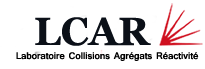# Laboratoire Collisions Agrégats Réactivité - UMR5589

Université Paul Sabatier - Bat. 3R1b4 - 118 route de Narbonne 31062 Toulouse Cedex 09, France

## Performance and application of a symmetry adapted pseudo spectral method for scattering of a diatomic molecule from a square surface : H2+Cu(111)

The H2 + Cu(1 0 0) system was used to test the performance of a newly developed, symmetry adapted pseudo spectral (SAPS) wave packet method for performing six dimensional quantum dynamical calculations on reactive scattering of diatomic molecules from square surfaces. Four test calculations have been performed on two different DFT PESs to compare the performance of the new SAPS method with that of the symmetry adapted wave packet (SAWP) method and the ordinary pseudo spectral method (PS). The comparison has been made for the (v=0,j=4,mj=0) and the (v=0,j=0,mj=0) initial states of H2, for the normal incidence energy ranges of 0.3–0.9 and 0.3–1.15 eV, respectively. The results for (v=0,j=4,mj=0) H2 reacting on PES 4, a PES expanded in a limited number of symmetry adapted functions so that it is suitable for use in the SAWP method, show that the SAPS method is competitive with the SAWP method, even for this PES. The results for (v=0,j=0,mj=0) H2 reacting on PES 5, a DFT PES represented using the corrugation reduction scheme, show that for this initial state the SAPS method is superior to the PS method by a factor of 6. It is concluded that, in general, for a given state the SAPS method will be at least three times faster than the PS method, also using at least three times less memory.

Voir en ligne : Chem. Phys. 304, 59–77 (2004)